The ACF is rather straightforward: we have a time series, and basically make multiple “copies” (as in “copy and paste”) of it, understanding that each copy is going to be offset by one entry from the prior copy, because the initial data contains $$t$$ data points, while the previous time series length (which excludes the last data point) is only $$t-1$$. We can make virtually as many copies as there are rows. Each copy is correlated to the original, keeping in mind that we need identical lengths, and to this end, we’ll have to keep on clipping the tail end of the initial data series to make them comparable. For instance, to correlate the initial data to $$ts_{t-3}$$ we’ll need to get rid of the last $$3$$ data points of the original time series (the first $$3$$ chronologically).

Example:

We’ll concoct a times series with a cyclical sine pattern superimposed on a trend line, and noise, and plot the R generated ACF. I got this example from an online post by Christoph Scherber, and just added the noise to it:

    set.seed(0)                          # To reproduce results.
x = seq(pi, 10 * pi, 0.1)            # Creating time-series as sin function.
y = 0.1 * x + sin(x) + rnorm(x)      # Time-series sinusoidal points with noise.
y = ts(y, start = 1800)              # Labeling time axis.
plot.ts(y)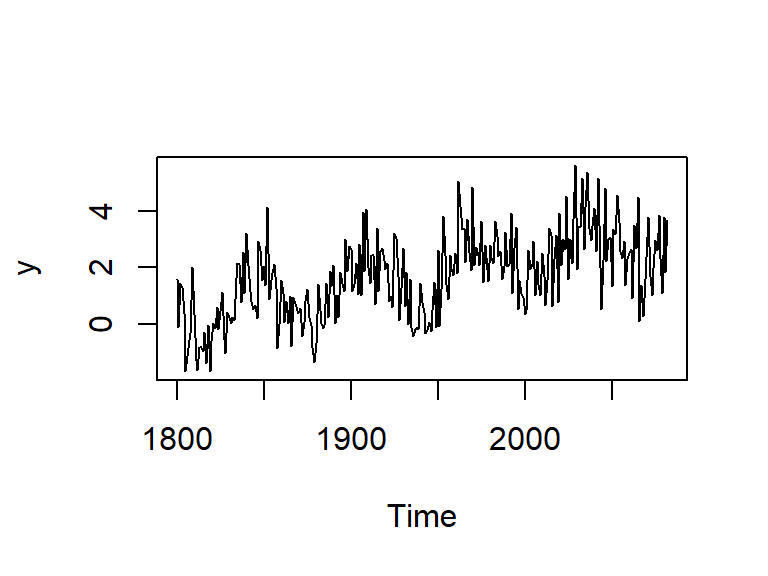Ordinarily we would have to test the data for stationarity (or just look at the plot above), but we know there is a trend in it, so let’s skip this part, and go directly to the de-trending step:

    model = lm(y ~ I(1801:2083))         # Detrending (0.1 * x)
st.y = y - predict(model)            # Final de-trended ts (st.y)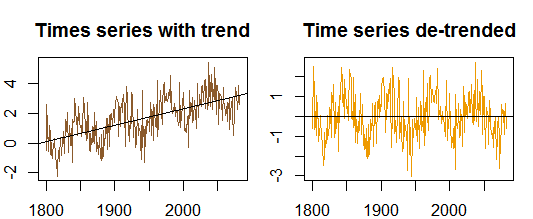Now we are ready to takle this time series by first generating the ACF with the acf() function in R, and then comparing the results to the makeshift loop I put together:

    cent = st.y - mean(st.y)                             # Centering the time series.
n = length(cent)                                     # Length of time series
ACF = 0                                              # Starting an empty vector to capture the auto-correlations.
z = sum(cent * cent) / n                             # Variance of time series.
ACF = z/z                                         # Normalizing first entry to 1.
for(i in 1:24){                                      # Took 24 points to parallel the output of acf()
lag = cent[-c(1:i)]                                  # Introducing lags in the stationary ts.
clipped = cent[1:(length(lag))]                      # Compensating by clipping the tail of the ts.
ACF[i + 1] = (sum(clipped * lag) / n) / z  # Storing each normalized correlation.
}
w = acf(st.y)                                        # Extracting values of acf without plotting
all.equal(ACF, as.vector(w$acf)) # TRUE ##  TRUE  par(mfrow = c(1,2)) acf(st.y, type = "correlation") # Plotting the built-in function (left) plot(ACF, type="h", main="ACF Manual calculation"); abline(h = 0) # and manual results (right). abline(h = c(1,-1) * 1.96/sqrt(length(st.y)), lty = 2, col="blue")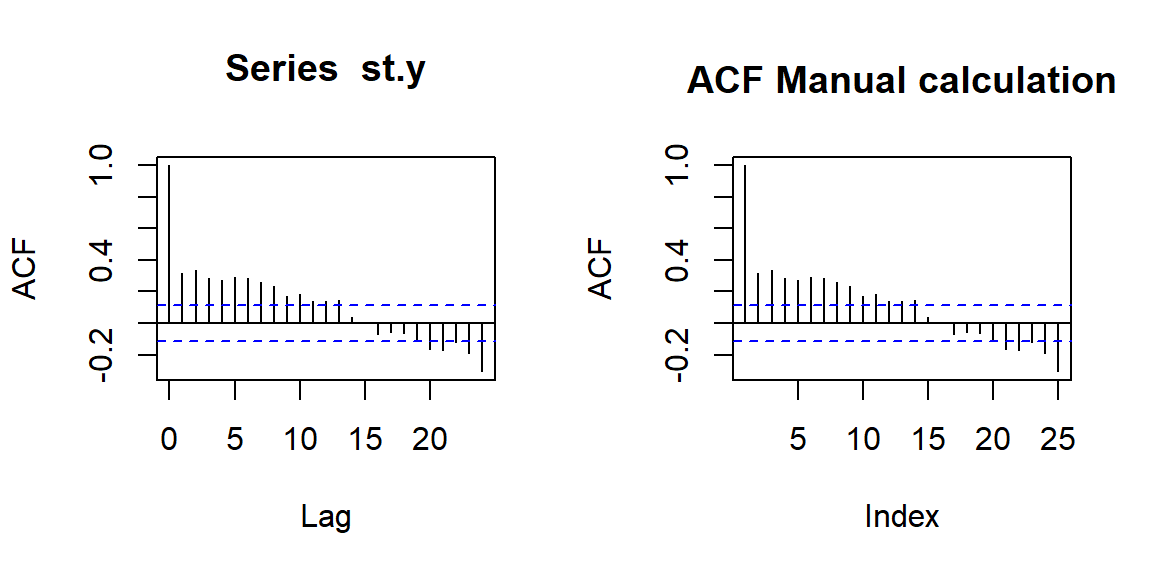Schematically, this is what we have done as: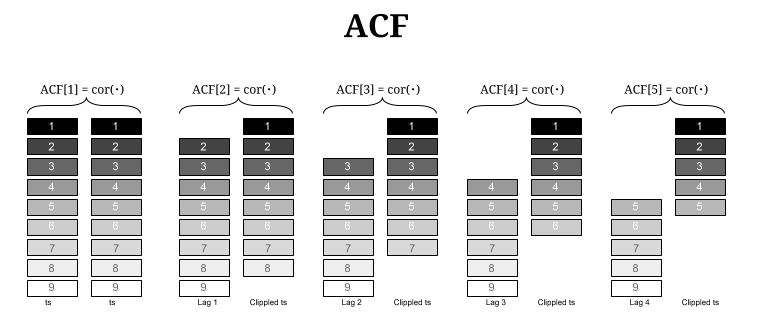##### Intution: For an MA model, the theoretical PACF does not shut off, but instead tapers toward 0 in some manner. A clearer pattern for an MA model is in the ACF. The ACF will have non-zero autocorrelations only at lags involved in the model. A moving average term in a time series model is a past error (multiplied by a coefficient). The $$q^{\text{th}}$$-order moving average model, denoted by MA(q) is $x_t = \mu + w_t +\theta_1w_{t-1}+\theta_2w_{t-2}+\dots + \theta_qw_{t-q}$ with $$w_t \overset{iid}{\sim} N(0, \sigma^2_w).$$ Here, it is not the message resemblance across time points that is searched backwards in time step-by-step, but rather the contribution of the noise, which I picture as the often massive deviations that a random walk can lead along the time line: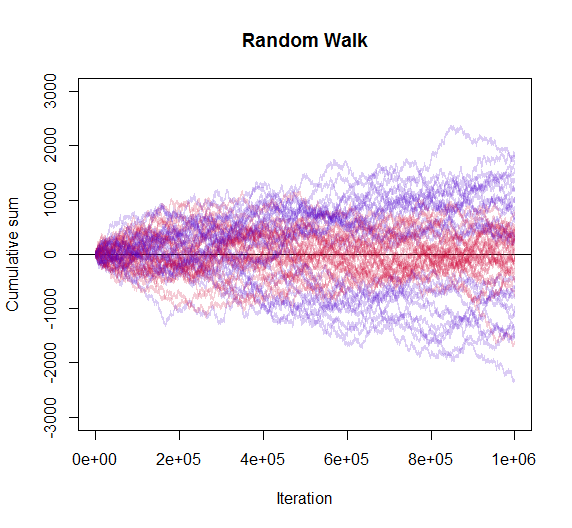Here there are multiple, progressively offset sequences that are correlated, discarding any contribution of the intermediate steps. In the telephone game analogy introduced below to explain PACF, “CV is cool!” is not completely different than “Naomi has a pool”. From the noise point of view, the rhymes are still there all the way to the beginning of the game. OK. That was successful. On to the PACF. Much more tricky to hack… The idea here is to again clone the initial ts a bunch of times, and then select multiple time points. However, instead of just correlating with the initial time series, we put together all the lags in-between, and perform a regression analysis, so that the variance explained by the previous time points can be excluded (controlled). For example, if we are focusing on the PACF ending at time $$ts_{t-4}$$, we keep $$ts_t$$, $$ts_{t-1}$$, $$ts_{t-2}$$ and $$ts_{t-3}$$, as well as $$ts_{t-4}$$, and we regress $$ts_t \sim ts_{t-1} + ts_{t-2} + ts_{t-3}+ts_{t-4}$$ through the origin and keeping only the coefficient for $$ts_{t-4}$$:  PACF = 0 # Starting up an empty storage vector. for(j in 2:25){ # Picked up 25 lag points to parallel R pacf() output. cols = j rows = length(st.y) - j + 1 # To end up with equal length vectors we clip. lag = matrix(0, rows, j) # The storage matrix for different groups of lagged vectors. for(i in 1:cols){ lag[ ,i] = st.y[i : (i + rows - 1)] # Clipping progressively to get lagged ts's. } lag = as.data.frame(lag) fit = lm(lag$V1 ~ . - 1, data = lag) # Running an OLS for every group.
PACF[j] = coef(fit)[j - 1]           # Getting the slope for the last lagged ts.
}
PACF = PACF[-1]                        # Getting rid of zero at the head of the vector
v = acf(st.y, type = "partial")        # PACF calculated by R
all.equal(PACF, as.vector(v\$acf))   #"Mean relative difference: 0.05580641"
##  "Mean relative difference: 0.05580641"
    par(mfrow = c(1,2))
pacf(st.y)
plot(PACF, type="h", main="PACF Manual calculation"); abline(h = 0) # and manual results (right).
abline(h = c(1,-1) * 1.96/sqrt(length(st.y)), lty = 2, col="blue")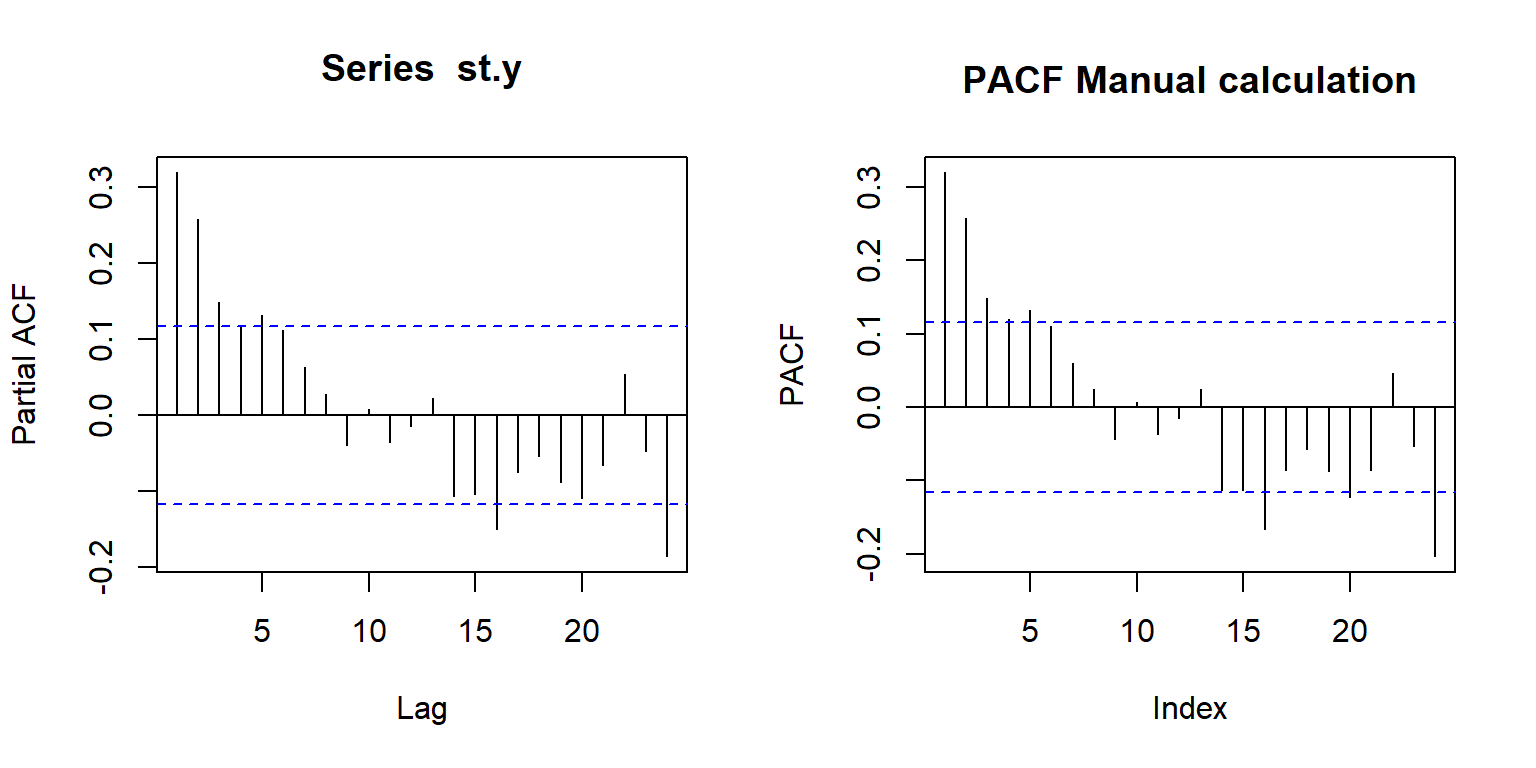Schematically, this is what we have done: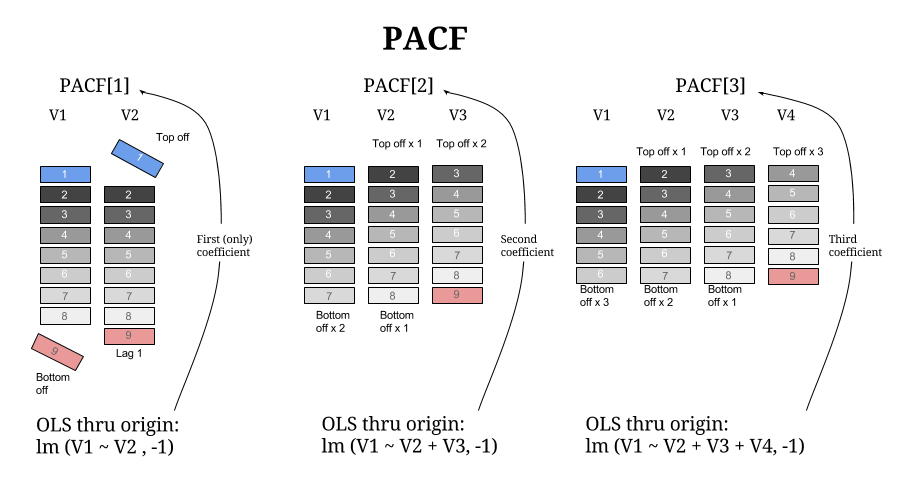That the idea is correct, beside probable computational issues, can be seen comparing PACF to pacf(st.y, plot = F).

##### Intuition:

Identification of an AR model is often best done with the PACF.

For an AR model, the theoretical PACF “shuts off” past the order of the model. The phrase “shuts off” means that in theory the partial autocorrelations are equal to 0 beyond that point. Put another way, the number of non-zero partial autocorrelations gives the order of the AR model. By the “order of the model” we mean the most extreme lag of x that is used as a predictor.

A $$k^{\text{th}}$$ order autoregression, written as AR(k), is a multiple linear regression in which the value of the series at any time t is a (linear) function of the values at times $$t-1,t-2,\ldots,t-k:$$

$y_{t}=\beta_{0}+\beta_{1}y_{t-1}+\beta_{2}y_{t-2}+\cdots+\beta_{2}y_{t-k}+\epsilon_{t}.$

This looks like a regression model.

What is a possible intuition of what we are doing here…

In Chinese whispers or the telephone game as illustrated here